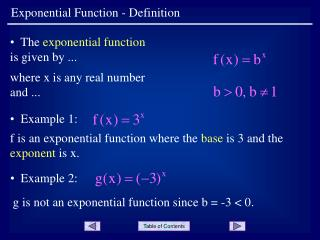DownloadDownload PresentationExponential Function - Definition

# Exponential Function - Definition

Download Presentation## Exponential Function - Definition

- - - - - - - - - - - - - - - - - - - - - - - - - - - E N D - - - - - - - - - - - - - - - - - - - - - - - - - - -
##### Presentation Transcript

1. Exponential Function - Definition • The exponential function is given by ... where x is any real number and ... • Example 1: f is an exponential function where the base is 3 and the exponent is x. • Example 2: g is not an exponential function since b = -3 < 0.

2. Exponential Function - Definition • Why is it necessary to have the restrictions on the base b? Consider the following examples. • Example 3: For all values of x, f(x) = 1. This reduces the equation to the simple linear equation, y = 1, which is not exponential. • Example 4: Using the table feature on a graphing calculator yields ... Slide 2

3. Exponential Function - Definition • Note that the data represents points on a graph that keep jumping from positive to negative to positive y-values. The points on the graph are not “connected” like most graphs are. • Another problem is what to do when x = 1/2, where which is complex. Slide 3

4. Exponential Function - Definition • Remember that the exponential function is quite different from a polynomial function. Polynomial Function Exponential Function     • The polynomial function has a variable base ... • The exponential function has a constant base ... and a constant exponent. and a variable exponent. Slide 4

5. Exponential Function - Definition END OF PRESENTATION Click to rerun the slideshow.